# Token Merging (ToMe) — Meta’s Optimization Technique to Make ViT Faster. But Can ViT be Even Faster?

Playing with ToMe and benchmarking it against other inference optimization strategies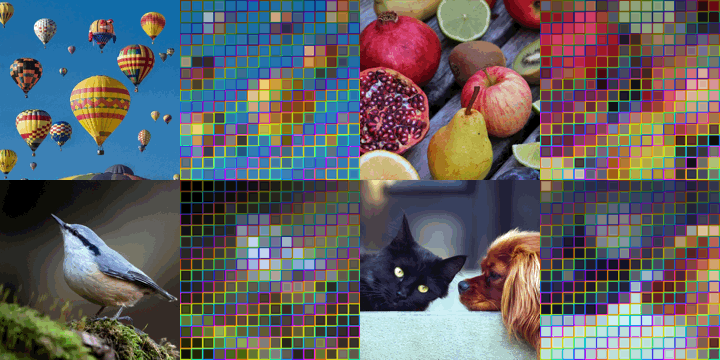Image taken from Meta’s blog: “Token Merging: Your ViT but faster”.

The goal of this blog is to explore Meta Research’s new Token Merging (ToMe) optimization strategy, perform some practical experiments with it, and benchmark ToMe with other state-of-the-art inference optimization techniques with the opensource library Speedster.

We will try to answer a few questions:

• What’s the accuracy-latency trade-off with ToMe?
• Can we reproduce Meta’s results?
• Meta tested Tome performances with an optimal batch size on a GPU V100. How does ToMe perform with different batch sizes and on cpus?
• How does ToMe perform compared to other optimization techniques, such as quantization or pruning and compilation?
• Can ToMe be combined with other optimization strategies to achieve a multiplier effect on throughput?
• Meta’s images are super cool 🙌. How can you ToMe-nize one of your pictures?

Let’s first explore ToMe.

## 🧩 ToMe: Token Merging

Token Merging (ToMe) is a technique recently introduced by Meta AI to reduce the latency of existing Vision Transformer (ViT) models without the need for additional training. ToMe gradually combines similar tokens into a transformer using an algorithm as lightweight as pruning while maintaining better accuracy.

ToMe introduces a module for token merging into an existing ViT, merging redundant tokens to improve both inference and training throughput.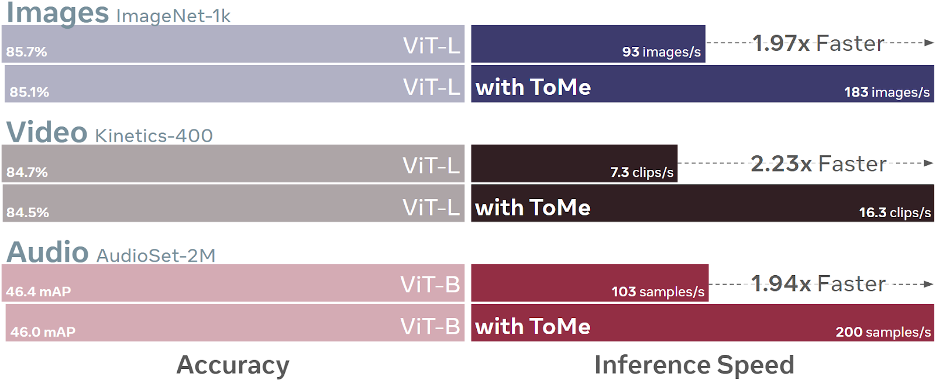ToMe accuracy vs inference speed performance. Image from Meta’s blog: “Token Merging: Your ViT but faster”.

## 🗺️ Optimization Strategy

ViT converts image patches into “tokens”. Then, it applies an attention mechanism to each layer that allows these tokens to collect information from one another proportionally to their similarity. To improve the speed of ViT while maintaining its accuracy, ToMe builds on two observations:

• Computation speed and memory use are heavily tied to the number of tokens in the transformer
• These tokens are often redundant

In each transformer block, tokens are combined (and thus reduced in number) by a quantity r of tokens per layer. Over the L blocks in the network, a number of rL tokens are merged. By varying the parameter r, we get a speed-accuracy trade-off, as fewer tokens means lower accuracy but higher throughput.

The image below shows how the token merging step is applied between the attention and MLP branches of each transformer block. Step by step, the dog’s fur is merged into a single token.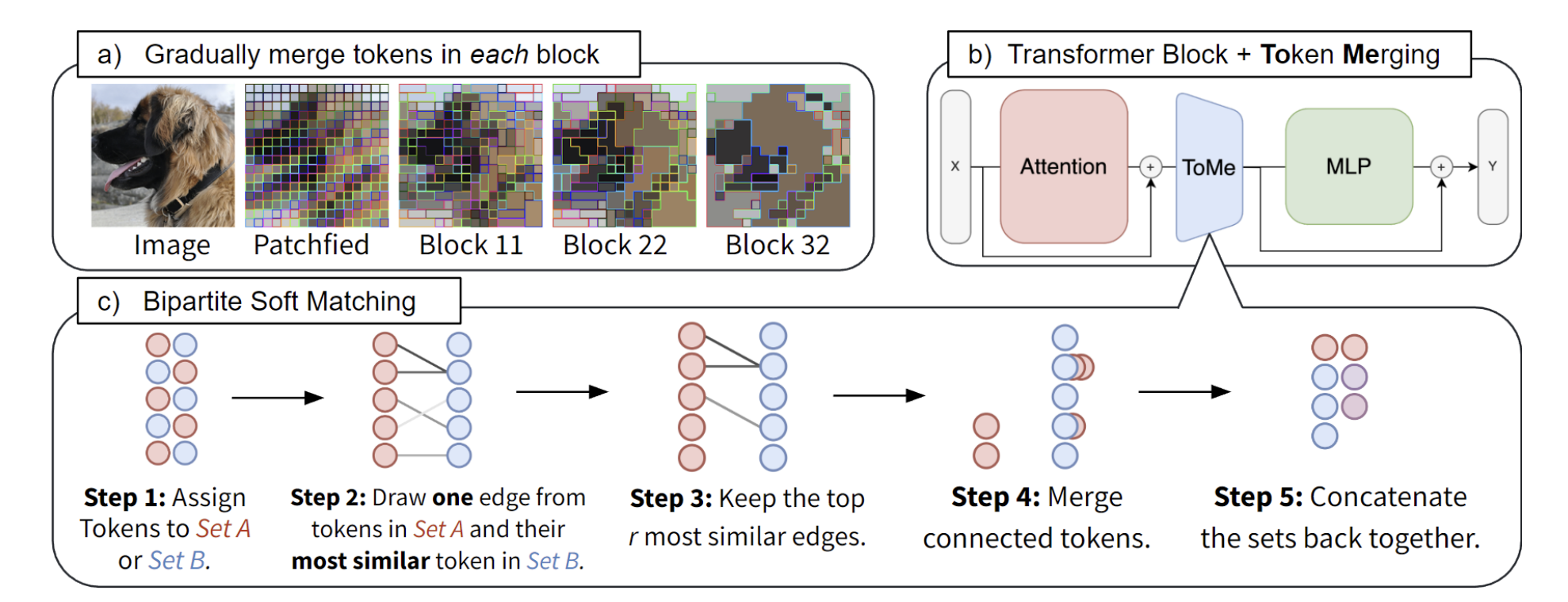Image taken from Meta’s paper: “Token Merging: Your ViT but faster”.

## 🤝 The Concept of Similarity

ToMe reduces the number of tokens by combining similar ones. The similarity between tokens is defined using self-attention QVKs. Specifically, the keys (K) summarise the information contained in each token. A dot product similarity metric (e.g. cosine similarity) between the keys of each token is then defined as a metric that measures the similarity between the different tokens, in order to understand whether they contain similar information.

## 📈 Paper Results

So, what’s the accuracy-latency trade-off with ToMe?

Let’s have a look at the results reported in the paper, which were obtained on a V100 GPU. I plotted the accuracy of ViT as a function of the hyperparameter r, where the original ViT corresponds to r=0. We can see that smaller values of r correspond to a model that is slower but with accuracy more faithful to the original model. Large values of r result in a considerable acceleration of the model but a loss in accuracy. For instance, to achieve 2x acceleration from the original model, the model loses 4 points of accuracy.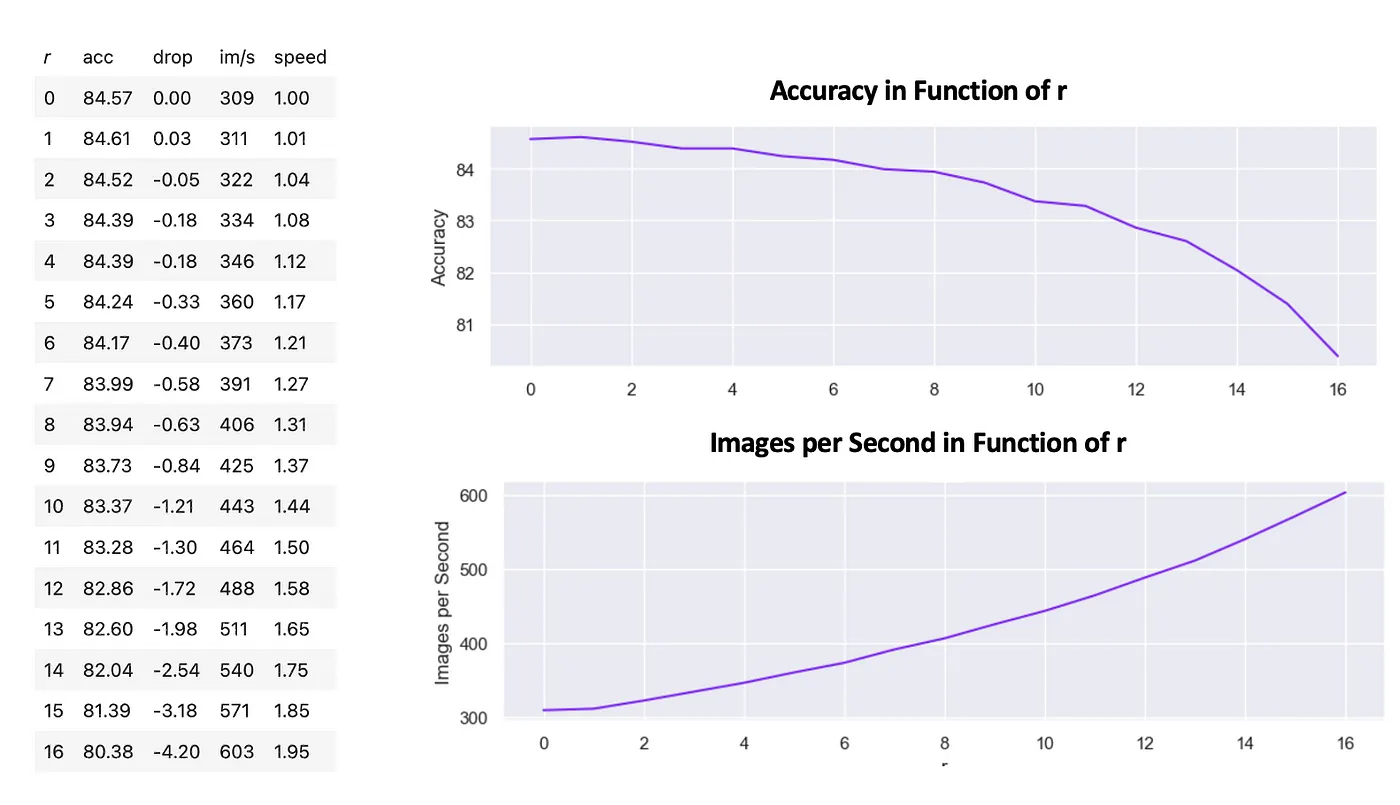Results in inference for the ViT-B/16 model.

All results shown are for the ViT-B/16 model, which is also the model that will be used in this notebook for the various experiments as well.

## 👷 Hands-on

Let’s see if we can reproduce Meta’s results.

I ran the experiments on ViT-B/16 model on a GPU V100 with batch size 64 as in the paper, the recommended value of the hyperparameter r=16. Then, I tested ToMe on smaller batch sizes up to batch size=1, and replicated the same experiment on a CPU E5–2686. A p3.2xlarge instance of aws was used for all experiments.

The results are very interesting:

• The use of ToMe is very simple, and we are able to reproduce Meta’s acceleration of 2x on a V100 for batch size 64.
• On GPU, ToMe is very sensitive to the batch size, and for low batch sizes it produces poorer performances with respect to the original model. This is because in this case the resources are not fully used when the batch size is small, in this case the highly parallelizable GPU still has space for further parallel computation. The token-reduction becomes significant on GPU only when the GPU power is fully utilized.
• On CPU, performances are around 2x both for very small and larger batch sizes. This is due to the fact that also for smaller batch sizes the CPU compute power is fully used by the network. Thus, the overhead of ToMe on CPU can already be compensated by the token-reduction for smaller batch sizes.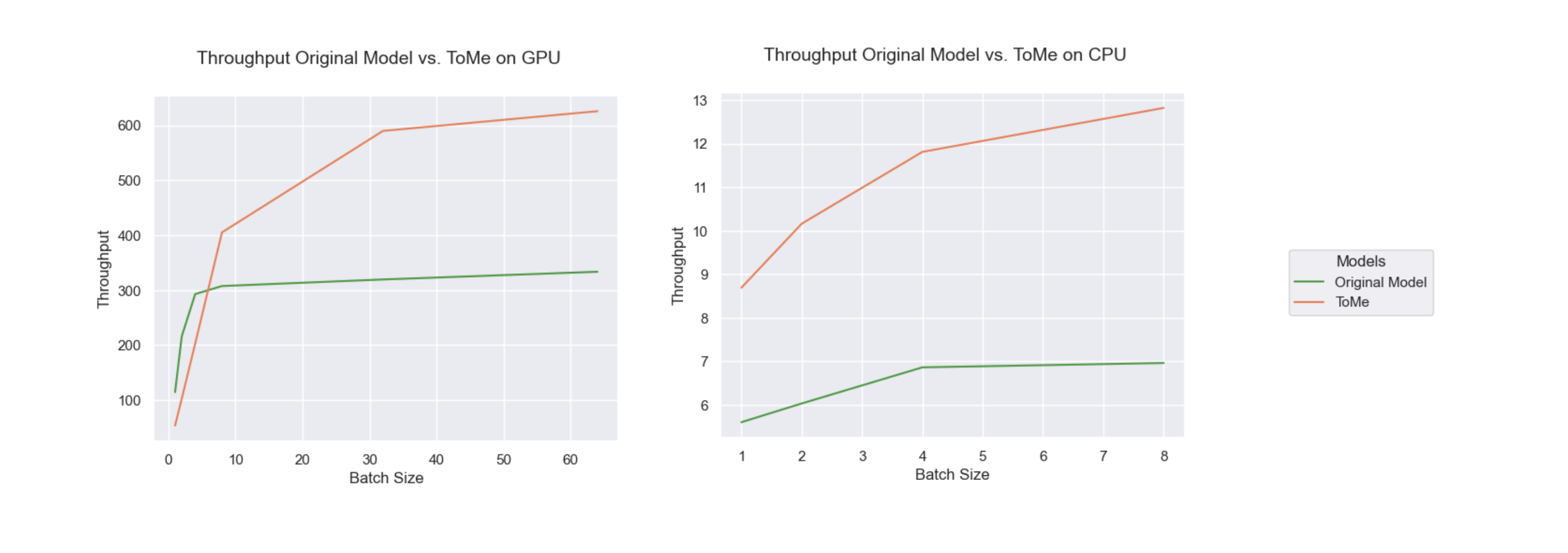Throughput graph for the original model and the model to which ToMe was applied, as the batch size varies.

Using ToMe is straightforward. Moreover, thanks to Nebullvm’s benchmark function, we can quickly evaluate the performance on both GPU and CPU of the original model and the optimized model:

``````
from nebullvm.tools.benchmark import benchmark

import timm, tome
import torch

# generate some input data (with batch size = 1 in this case)
input_data = [((torch.randn(1, 3, 224, 224), ), torch.tensor()) for _ in range(100)]

model = timm.create_model("vit_base_patch16_224", pretrained=True)
model.eval()

# benchmark of the original model on gpu
benchmark(model, input_data)
# benchmark of the original model on cpu
# for the cpu, I lowered the warmup value (set to 50 by default)
benchmark(model, input_data, device="cpu", n_warmup=20, n_runs=100)

# ToMe optimization
tome.patch.timm(model)
# Set the number of tokens reduced per layer
model.r = 16

# benchmark of the optimized model on gpu
benchmark(model, input_data)
# benchmark of the optimized model on cpu
benchmark(model, input_data, device="cpu", n_warmup=20, n_runs=100)
```
```

#### 🚀 ToMe vs Other Optimization Techniques

As a next step, I compared the performance of ToMe with what can be achieved by other optimization techniques. I used the Speedster library to run the optimizations and see the performance on CPU and GPU.

Speedster is an open-source module designed to speed up AI inference in just a few lines of code. Its use is very simple. The library automatically applies the best set of SOTA optimization techniques to achieve the maximum inference speed-up (of latency and throughput, while compressing the model size) physically possible on the available hardware.

The optimization workflow consists of 3 steps: select, search, and serve.

📚 Select step: in this step, users input their model in their preferred deep learning framework and express their preferences regarding maximum consented accuracy loss and optimization time. This information is used to guide the optimization process and ensure that the resulting model meets the user’s needs.

🔍 Search step: the library automatically tests every combination of optimization techniques across the software-to-hardware stack, such as sparsity, quantization, and compilers, that is compatible with the user’s preferences and local hardware. This allows the library to find the optimal configuration of techniques for accelerating the model.

🍵 Serve step: in this final step, the library returns an accelerated version of the user’s model in the DL framework of choice, providing a significant boost in performance.

The model is optimized by the 4 Speedster blocks shown in the image below. How they work is presented in the library documentation.Image taken from Speedster documentation.

#### 💫 ViT Optimization with Speedster

I performed two experiments with Speedster. First, I performed only optimization with techniques that have no impact on model performance. This is achieved by setting the parameter metric_drop_ths=0.

Next, I increased the metric_drop threshold to 0.05 so that speedster could also apply techniques that slightly change the accuracy to provide better speedup, such as quantization and compression. The 0.05 value is very low, which means that we expect the accuracy to remain essentially unchanged, as explained in the documentation.

Let’s analyze the results:

• Speedster is easy to use and allows information to be obtained during optimization regarding the techniques tested.
• The library makes it possible to display initial metrics related to model acceleration without the need to run the benchmark function.
• Using half precision, flash attention and hardware-specific compilation techniques, Speedster on GPU can significantly accelerate the model with a target metric lower than 0.05 compared to the original version. The default metric used by speedster is the numeric precision, which measures the average relative difference between the original model and the optimized one. Therefore, any model with a target metric lower than 0.05 can be considered as having no accuracy loss.
• The original model and Speedster had similar throughput on CPU. Increasing the metric drop to 0.05 did not help with speed-up. This is because many layers in Speedster do not have fp16 kernels, so converting fp32 tensors to fp16 and back inside the network slows down latency. Also, int8 conversion for the ViT model made numeric precision drop, and did not meet the 0.05 constraint. This makes sense because transformers are more affected by quantization, due to having “outliers” in the activations. Using QAT before quantizing, i.e. fine-tuning the model with simulated quantization, can then be used for getting a better speed-up with Speedster on CPUs. One way to improve Speedster performance on CPUs might be to implement ToMe within the library.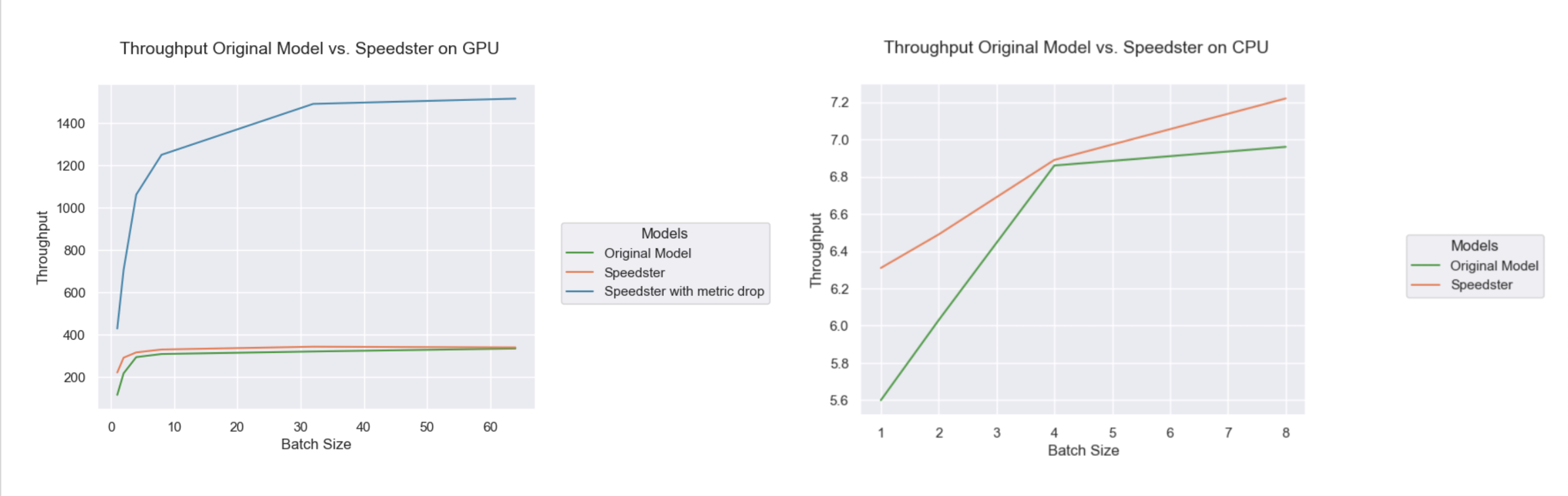Throughput graph for the original model and the model to which Speedster was applied, as the batch size varies.

Using Speedster is very simple, and again performance is measured using the benchmark function. Optimization performance is also automatically displayed in the logs when you run the optimization.

``````
# generate some input data (with batch size = 1 in this case)
input_data = [((torch.randn(1, 3, 224, 224), ), torch.tensor()) for _ in range(100)]

model = timm.create_model("vit_base_patch16_224", pretrained=True)
model.eval()

# Speedster optimization without metric drop
optimized_model = optimize_model(
model,
input_data=input_data,
optimization_time="constrained",
metric_drop_ths=0
)

# Speedster optimization with an acceptable metric loss of 10%
optimized_model_drop = optimize_model(
model,
input_data=input_data,
optimization_time="constrained",
metric_drop_ths=0.05
)

# benchmark of the optimized model without metric drop on gpu
benchmark(optimized_model, input_data)
# benchmark of the optimized model without metric drop on cpu
benchmark(optimized_model, input_data, device="cpu", n_warmup=20, n_runs=100)

# benchmark of the optimized model with metric drop on gpu
benchmark(optimized_model_drop, input_data)
# benchmark of the optimized model with metric drop on cpu
benchmark(optimized_model_drop, input_data, device="cpu", n_warmup=20, n_runs=100)
```
```

#### 🎨 Test ToMe With Your Own Picture

In the notebook you can find a section where you can test ToMe on your images, with the possibility of changing the hyperparameter r that adjusts the level of optimization. I did some testing and I felt 100% in the Infinity War movie: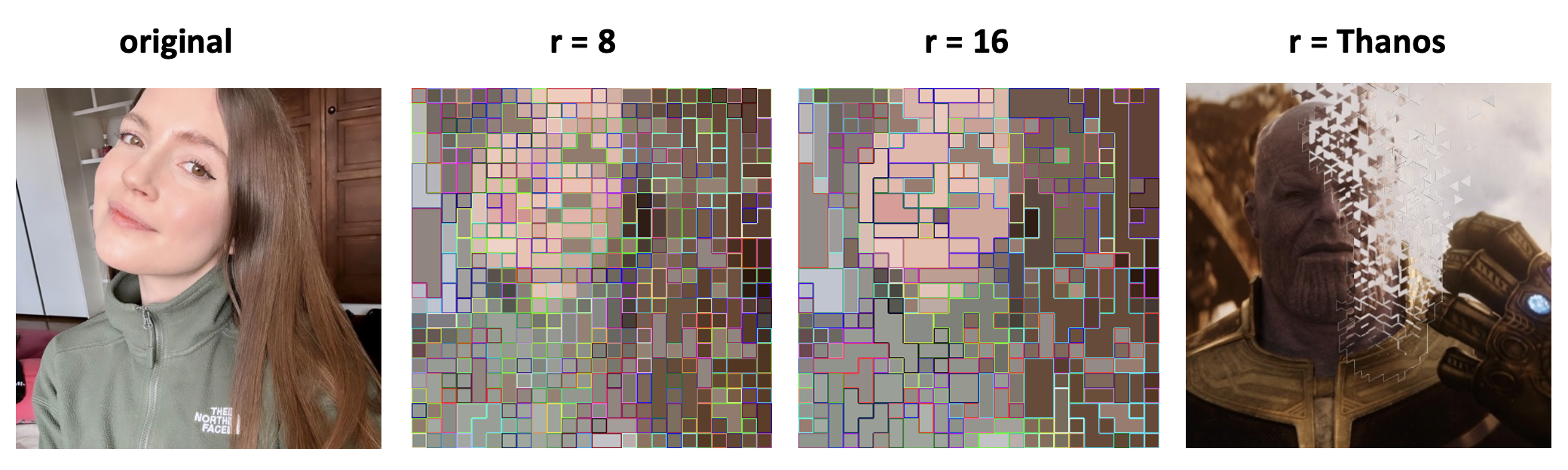ToMe test on a picture of me.

This experiment can be done by preprocessing your image and using ToMe’s make_visualization method:

``````
from torchvision import transforms
from torchvision.transforms.functional import InterpolationMode
from PIL import Image

model_name = "vit_large_patch16_384"
model = timm.create_model(model_name, pretrained=True)

# apply tome
tome.patch.timm(model, trace_source=True)

# image preprocessing
input_size = model.default_cfg["input_size"]

transform_list = [
transforms.Resize(int((256 / 224) * input_size), interpolation=InterpolationMode.BICUBIC),
transforms.CenterCrop(input_size)
]

transform_vis  = transforms.Compose(transform_list)
transform_norm = transforms.Compose(transform_list + [
transforms.ToTensor(),
transforms.Normalize(model.default_cfg["mean"], model.default_cfg["std"]),
])

# change this with the path of your image!
PATH_TO_IMAGE = "imgs/img.jpg"

img = Image.open(PATH_TO_IMAGE).convert('RGB')

img_vis = transform_vis(img)
img_norm = transform_norm(img)

# here you can change the hyper-parameter r:
model.r = 16
_ = model(img_norm[None, ...])
source = model._tome_info["source"]

print(f"{source.shape} tokens at the end")
tome.make_visualization(img_vis, source, patch_size=16, class_token=True)
```
```

#### 🌈 Conclusions

ToMe makes it possible to accelerate Visual Transformer models, both on GPU and CPU. One interesting thing to notice is that ToMe improves the model’s speed on CPU inference, but reduces it on GPU when the batch size is low. This can be explained by the fact that CPU uses its full compute power for smaller batch sizes, while GPU has more room for parallel computation. Therefore, ToMe’s overhead on CPU is offset by the token reduction, but not on GPU until the batch size is large enough. This can also be seen from the graphs below: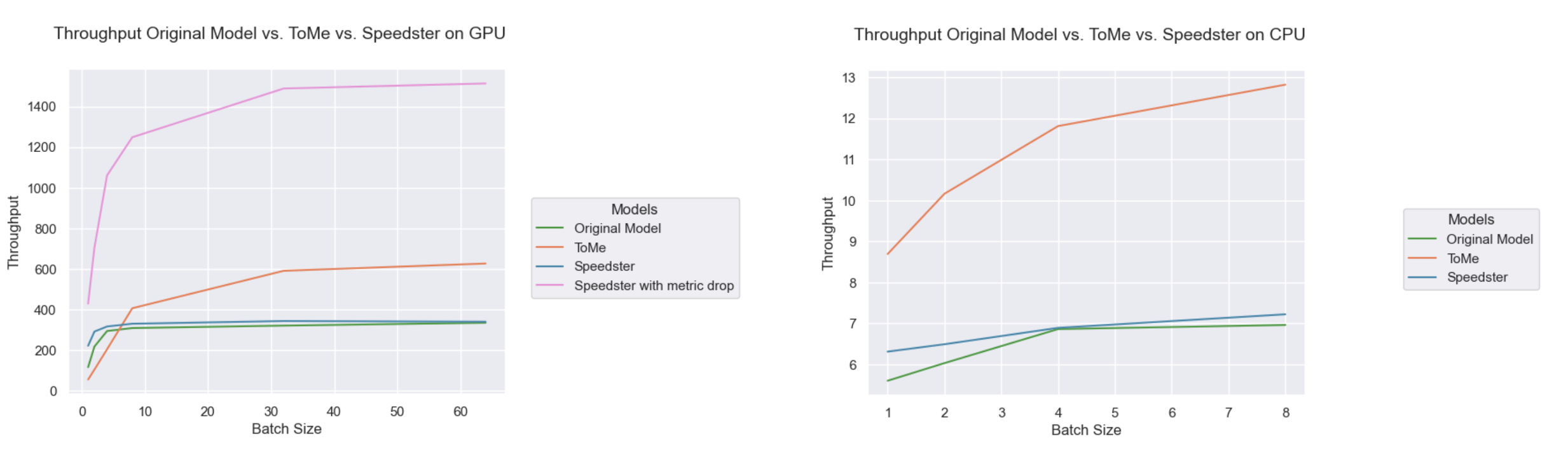Results obtained with different optimization techniques, with various values for batch size.

Here we can see that Speedster accepting a 5% performance loss is significantly faster than the original model, remembering that ToMe also leads to performance losses the comparison between the techniques can be considered fair. While on the CPU ToMe appears to be the fastest technique, so it might be interesting to implement its automatic use within Speedster. I opened an issue on Speedster GitHub so that anyone can contribute.

And that’s it! If you are interested in AI optimization or if you liked this notebook please leave a star at our repo Speedster 💕🌟!

#### 💾 References

Do you also want to play with ToMe? I have prepared a notebook very similar to this blog, where you can also test ToMe by yourself… And get beautiful pictures with ToMe 💁